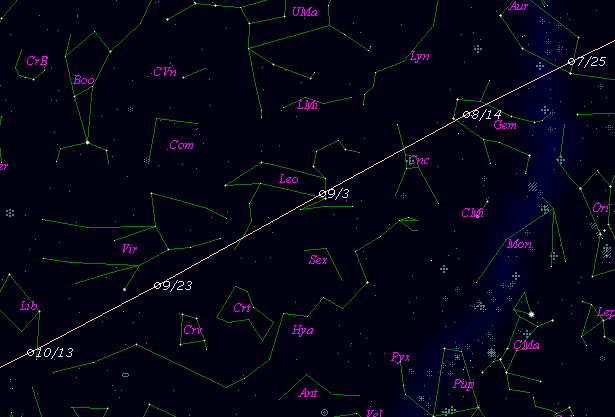# 2P/Encke (2000)###Orbital Elements

```Epoch 2000 Sept. 13.0 TT = JDT 2451800.5
T 2000 Sept. 9.66111 TT                                 Muraoka
q   0.3395369            (2000.0)            P               Q
n   0.29843017     Peri.  186.48275     -0.94497665     -0.31524986
a   2.2177244      Node   334.60082     +0.30855015     -0.77013416
e   0.8468985      Incl.   11.75566     +0.10870110     -0.55453666
P   3.30
>From 165 observations 1982-1995, mean residual 0".89.  Nongravitational
parameters A1 = -0.01 +/- 0.00, A2 = -0.0017 +/- 0.0000.
```

###Finding Charts###Magnitudes Graph

```        H = 14.2  G = 0.15                [    ,-100]  (             - 2000 June  1)
m1 =  9.8 + 5 log d + 25   log r  [-100, -45]  (2000 June  1 - 2000 July 26)
m1 = 10.3 + 5 log d +  7.0 log r  [ -45,  20]  (2000 July 26 - 2000 Sept.29)
m1 = 12.3 + 5 log d + 15.7 log r  [  20, 100]  (2000 Sept.29 - 2000 Dec. 18)
H = 14.2  G = 0.15                [ 100,    ]  (2000 Dec. 18 -             )

* Green curve is:  H = 14.2  G = 0.15
```##### The orbital elements are calculated by Kenji Muraoka. The charts are made with StellaNavigator Ver.2.0 for Windows (AstroArts / ASCII). The magnitudes graphs are made with Comet for Windows.